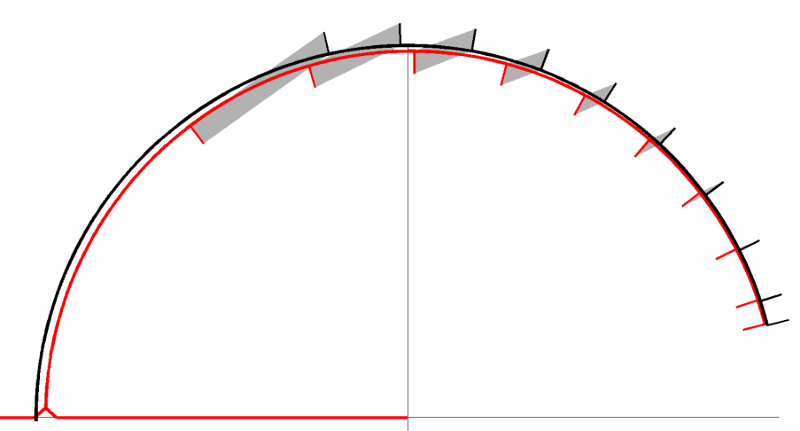# Baroclinic Momentum Equations¶

## Baroclinic Momentum Equations¶

The baroclinic momentum equations are the stacked shallow water equations:

$\frac{\partial \vec{u}_k}{\partial t} + (f + \nabla_s \times \vec{u}_k) \hat{z} \times \vec{u}_k = - \frac{\nabla_s p_k}{\rho} - \nabla_s (\phi_k + \frac{1}{2} || \vec{u}_k ||^2 ) + \frac{\nabla \cdot \tilde{\tau}_k}{\rho}$
$\frac{\partial h_k}{\partial t} + \nabla_s \cdot (\vec{u}h_k) = 0$

The timestepping for these equations is a (quasi?) second-order Runge-Kutta step for the inertial oscillations and a forward-backward Euler step for the pressure (gravity) waves. Using the graphical notation from , it looks like:Graphical notation for timestepping schemes in which the black line represents the ideal solution and the red line shows the actual solution. Phase errors are represented by the grey shapes between the bars normal to the circle.¶

The timestep used in ROMS looks instead like: